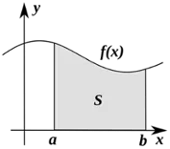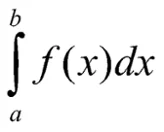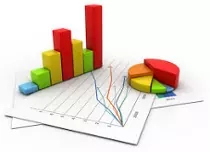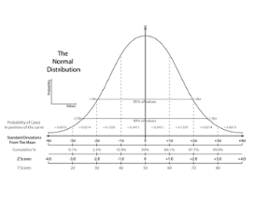# Calculus v. Statistics is it an Ongoing Battle?

Which is more useful? Which is more significant to science and business or civilization?

I believe calculus has to come first but I don’t really think either is better.  This is my opinion. Both have their own appropriate applications and end goals.

Just a quick high-level review and opinion refresher here…

Calculus is Deterministic, if you need to plot a course to Mars, you would use calculus.

Statistics is Relativistic. Statistics would be used more to decide where you would want to land on Mars.Vs.According to Wikipedia Calculus is the mathematical study of change as Geometry is the study of shape and algebra is the study of operations and their application to solving equations.

The study of Calculus has two major branches; differential calculus (rates of change and slopes of curves) and integral calculus (accumulation and area under a curve)

Statistics on the other hand is about the collection, all aspects of analysis, interpretation, presentation, and organization of data.

The two main branches of statistics are  1) Descriptive statistics which is about quantitatively describing or summarizing the main features of a collection of information or the quantitative description of the data itself.  Concepts like the mean and the standard deviation are the realm of Descriptive Statistics. and 2) Inferential statistics are used to infer properties about a population of data: this includes testing hypotheses and deriving estimates. The population is assumed to be larger than the observed data set; in other words, the observed data is assumed to be sampled from a larger population which attempts to infer propositions.  Point estimates and interval estimates are the realms of Inferential Statistics, according to Wikipedia, interval (Statistical) estimation is the use of sample data to calculate an interval of possible (or probable) values of an unknown population parameter, in contrast to point estimation, which is a single number.

Calculus is used in every branch of science, computer science, (even) statistics, engineering, economics, business, medicine, and in other fields wherever a problem can be mathematically modeled and an optimal solution is desired. It allows one to go from (non-constant) rates of change to the total change or vice versa. Often studying a problem where we know one and are trying to find the other related data.

Statistics helps us find truths or trends in the mountains of data we produce, often reducing the need to do Calculus on each and every alternate possibility.

People often lean towards one or the other as a preference but I think there isn’t really a battle of one being better than the other.

Personally, I prefer Calculus for its deterministic nature. Maybe also due to being exposed to more of it in school and work. If I had to choose one it would be Calculus.

As a final comparison on the more practical level in my work, Calculus is used to design the circuit; Statistics are used to determine the most effective testing of the product functionality and reliability before shipment.

Both apply if you are doing product testing.  If you are thermal testing in temperature chambers or on a thermal platform, you might be verifying a design built on Calculus or using Statistics to ask the questions and get answers about the yield on your production.